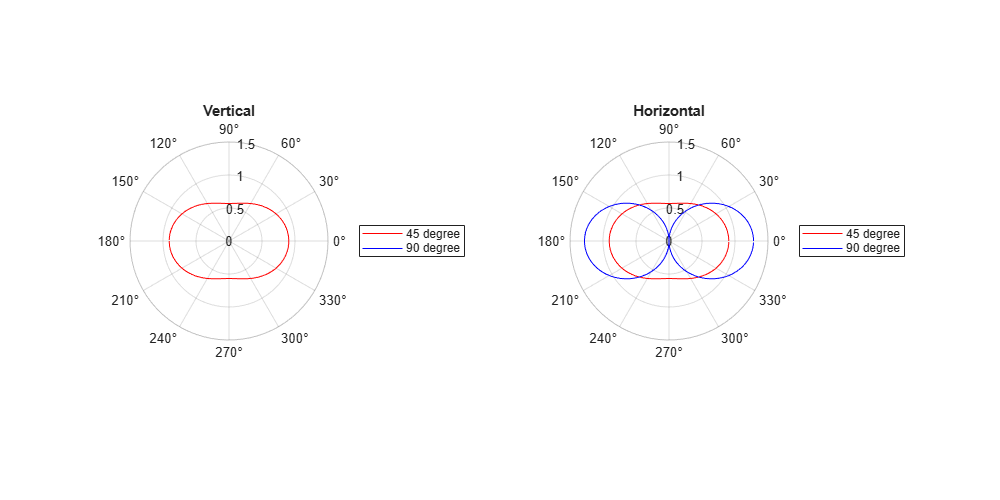# winner2.dipole

Calculate field pattern of half-wavelength dipole

## Syntax

``pat = winner2.dipole(az)``
``pat = winner2.dipole(az,slant)``

## Description

````pat = winner2.dipole(az)` returns the azimuth field pattern of a 0-degree slanted dipole at the azimuth angles specified in `az`.```

example

````pat = winner2.dipole(az,slant)` returns the azimuth field pattern of a slanted dipole at the azimuth angles specified in `az`.```

## Examples

collapse all

Create 45 and 90 degree slanted dipoles by using the `winner2.dipole` function.

```az = -180:179; % 1 degree spacing pattern45 = squeeze(winner2.dipole(az,45)); pattern90 = squeeze(winner2.dipole(az,90));```

Display the antenna pattern by using the `polarplot` function.

```fh = figure; set(fh, 'Position', [100 100 1000 500]); fh.Name = 'Dipole Pattern Plots'; subplot(1,2,1); polarplot(az/180*pi,pattern45(1,:),'r'); hold on; polarplot(az/180*pi,pattern90(1,:),'b'); rlim([0 1.5]); legend('45 degree','90 degree'); title('Vertical'); subplot(1,2,2); polarplot(az/180*pi,pattern45(2,:),'r'); hold on; polarplot(az/180*pi,pattern90(2,:),'b'); rlim([0 1.5]); legend('45 degree','90 degree'); title('Horizontal');```## Input Arguments

collapse all

Azimuth angles, specified as a vector indicating the azimuth angles to compute the field pattern gain. Units are in degrees.

Data Types: `double`

Slant angle, specified as a scalar representing the counterclockwise angle seen from the front of the dipole. Units are in degrees.

Data Types: `double`

## Output Arguments

collapse all

Field pattern, returned as a 2-by-1-by-NAZ array representing the vertical and horizontal field pattern, where NAZ is the number of elements in the `az` input vector.

 Kyosti, Pekka, Juha Meinila, et al. WINNER II Channel Models. D1.1.2 V1.2. IST-4-027756 WINNER II, September 2007.